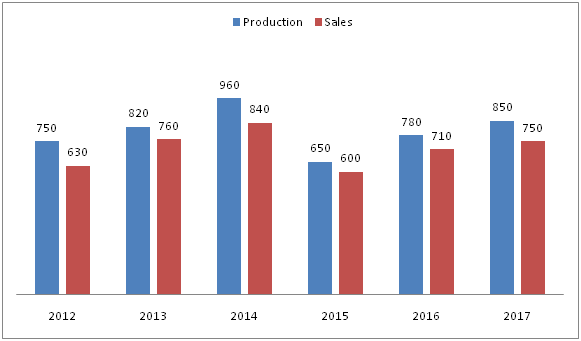# IBPS Clerk Prelims 2018 – Quantitative Aptitude Questions Day-29

Dear Readers, Bank Exam Race for the Year 2018 is already started, To enrich your preparation here we have providing new series of Practice Questions on Quantitative Aptitude – Section. Candidates those who are preparing for IBPS Clerk Prelims 2018 Exams can practice these questions daily and make your preparation effective.

[WpProQuiz 4307]

Directions (Q. 1 – 5): What value should come in place of question mark (?) in the following questions?

1) 45 % of 600 – 18 % of 350 =? – 62 – 252

a) 728

b) 756

c) 894

d) 912

e) None of these

2) 4 2/3 ÷ (6/11) × (?/22) = 4 1/4 × (7/9) × (3/14)

a) 51/28

b) 47/32

c) 33/16

d) 78/43

e) None of these

3) ? = (3745 + 1780) ÷ 17 + 162 + (5/11) of 880 – 15

a) 824

b) 872

c) 938

d) 966

e) None of these

4) 2516 × ? + 16 × 11 = 2526 + 5198

a) 5

b) 3

c) 7

d) 9

e) None of these

5) 156 ÷ 13 + 19683 – 12 × 15 =? – 83

a) 432

b) 415

c) 371

d) 384

e) None of these

Directions (Q. 6 – 10) Study the following information carefully and answer the given questions:

The following bar graph shows the total production and total sales (In tonnes) of a certain item in a company in different years.6) Find the difference between total production in the year 2012 and 2015 together to that of total sales in the year 2013 and 2016 together?

a) 90 tonnes

b) 110 tonnes

c) 70 tonnes

d) 125 tonnes

e) None of these

7) Find the average number of items remained in stock in the year 2013, 2015 and 2017 together?

a) 70 tonnes

b) 85 tonnes

c) 60 tonnes

d) 76 tonnes

e) None of these

8) Find the ratio between the total sales in the year 2012 and 2014 together to that of total production in the year 2013 and 2016 together?

a) 82: 115

b) 43: 57

c) 12: 29

d) 147: 160

e) None of these

9) The total production and sales in the year 2012 is what percentage of total production and sales in the year 2015?

a) 96.5 %

b) 110.4 %

c) 88.3 %

d) 72.6 %

e) None of these

10) Total sales in all the given years together is approximately what percentage more/less than the total production in all the given years together?

a) 3 % less

b) 3 % more

c) 11 % less

d) 20 % more

e) 11 % more

Direction (1-5 ) :

45 % of 600 – 18 % of 350 = x – 62 – 252

(45/100)*600 – (18/100)*350 + 62 + 625 = x

X = 270 – 63 + 62 + 625

X = 894

4 2/3 ÷ (6/11) × (x/22) = 4 1/4 × (7/9) × (3/14)

(14/3)*(11/6)*(x/22) = (17/4)*(7/9)*(3/14)

X = 51/28

x = (3745 + 1780) ÷ 17 + 162 + (5/11) of 880 – 15

x = 5525/17 + 256 + (5/11)*880 – 15

x = 325 + 256 + 400 – 15

x = 966

2516 × x + 16 × 11 = 2526 + 5198

2516 × x + 176 = 7724

2516 × x = 7548

X = 7548/2516 = 3

156 ÷ 13 + ∛19683 – 12 × 15 = x – 83

(156/13) + 27 – (12*15) + 512 = x

X = 12 + 27 – 180 + 512

X = 371

Direction (6-10) :

The total production in the year 2012 and 2015 together

= > 750 + 650 = 1400 tonnes

The total sales in the year 2013 and 2016 together

= > 760 + 710 = 1470 tonnes

Required difference = 1470 – 1400 = 70 tonnes

The total number of items remained in stock in the year 2013, 2015 and 2017 together

= > (820 – 760) + (650 – 600) + (850 – 750)

= > 60 + 50 + 100 = 210 tonnes

Required average = 210/3 = 70 tonnes

The total sales in the year 2012 and 2014 together

= > 630 + 840 = 1470 tonnes

The total production in the year 2013 and 2016 together

= > 820 + 780 = 1600 tonnes

Required ratio = 1470: 1600 = 147: 160

The total production and sales in the year 2012

= > 750 + 630 = 1380 tonnes

The total production and sales in the year 2015

= > 650 + 600 = 1250 tonnes

Required % = (1380/1250)*100 = 110.4 %

Total sales in all the given years together

= > 630 + 760 + 840 + 600 + 710 + 750 = 4290

Total production in all the given years together

= > 750 + 820 + 960 + 650 + 780 + 850 = 4810

Required % = [(4810 – 4290)/4810]*100 = 11 % less

Daily Practice Test Schedule | Good Luck

 Topic Daily Publishing Time Daily News Papers & Editorials 8.00 AM Current Affairs Quiz 9.00 AM Current Affairs Quiz (Hindi) 9.30 AM IBPS Clerk Prelims – Reasoning 10.00 AM IBPS Clerk Prelims – Reasoning (Hindi) 10.30 AM IBPS Clerk Prelims – Quantitative Aptitude 11.00 AM IBPS Clerk Prelims – Quantitative Aptitude (Hindi) 11.30 AM Vocabulary (Based on The Hindu) 12.00 PM IBPS PO Prelims – English Language 1.00 PM SSC Practice Questions (Reasoning/Quantitative aptitude) 2.00 PM IBPS PO/Clerk – GK Questions 3.00 PM SSC Practice Questions (English/General Knowledge) 4.00 PM Daily Current Affairs Updates 5.00 PM IBPS PO Mains – Reasoning 6.00 PM IBPS PO Mains – Quantitative Aptitude 7.00 PM IBPS PO Mains – English Language 8.00 PM